# Introduction to Algebra

Algebra is great fun – you get to solve puzzles!

## A Puzzle

What is the missing number?

2 = 4

OK, the answer is 6, right? Because 6 − 2 = 4. Easy stuff.

Well, in Algebra we don’t use blank boxes, we use a letter (usually an x or y, but any letter is fine). So we write:

 x − 2 = 4

It is really that simple. The letter (in this case an x) just means “we don’t know this yet”, and is often called the unknown or the variable.

And when we solve it we write:

 x = 6

## Why Use a Letter?

 Because:it is easier to write “x” than drawing empty boxes (and easier to say “x” than “the empty box”).if there are several empty boxes (several “unknowns”) we can use a different letter for each one.

So x is simply better than having an empty box. We aren’t trying to make words with it!

And it doesn’t have to be x, it could be y or w … or any letter or symbol we like.

## How to Solve

Algebra is just like a puzzle where we start with something like “x − 2 = 4” and we want to end up with something like “x = 6”.

But instead of saying “obviously x=6″, use this neat step-by-step approach:

• Work out what to remove to get “x = …”
• Remove it by doing the opposite (adding is the opposite of subtracting)
• Do that to both sides

Here is an example:

We want
to remove
the “−2”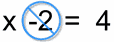To remove it, do
the opposite
, in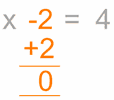Do it to
both sides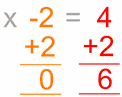Which is …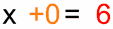Solved!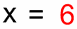## Why did we add 2 to both sides?

### To “keep the balance”…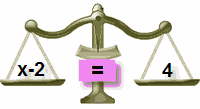In Balance
 Add 2 to Left SideOut of Balance!
 Add 2 to Right Side Also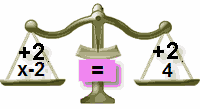In Balance Again

Just remember this:

 To keep the balance, what we do to one side of the “=” we should also do to the other side!

See this in action at the Algebra Balance Animation.

# Solve this one:

 x + 5 = 12

What we want is an answer like “x = …”,
but the +5 is in the way of that!
We can cancel out the +5 with a −5 (because 5−5=0)

So, let us have a go at subtracting 5 from both sides:x + 5 −5 = 12 −5
A little arithmetic (5−5 = 0 and 12−5 = 7) becomes:x + 0 = 7
Which is just:x = 7
Solved!
(Quick Check: 7+5=12)

## Have a Try Yourself

Now practice on this Simple Algebra Worksheet and then check your answers. Try to use the steps we have shown you here, rather than just guessing!

Try the questions below, then read Introduction to Algebra – Multiplication